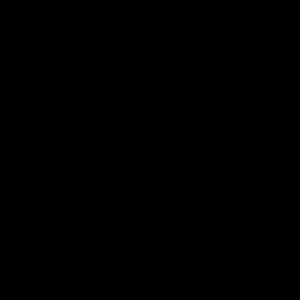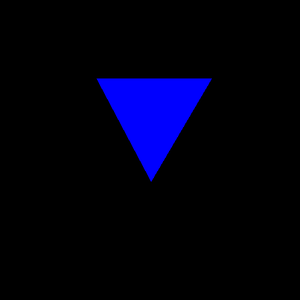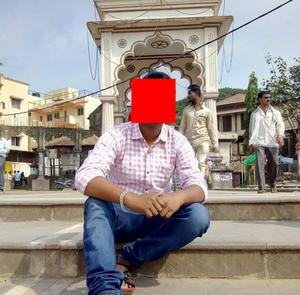GeeksforGeeks App
Open AppBrowser
Continue

# Draw a filled polygon using the OpenCV function fillPoly()

fillPoly() function of OpenCV is used to draw filled polygons like rectangle, triangle, pentagon over an image. This function takes inputs of an image and endpoints of Polygon and color.

Syntax: cv2.fillpoly(Image,End_Points,Color)

Parameter:

• Image: This is image on which we want draw filled polygon
• End_Points: Points of polygon(for triangle 3 end points, for rectangle 4 end points will be there)
• Color: It specifies the color of polygon

### Example 1: Draw a triangle

In this example we will draw filled polygon triangle by giving 3 endpoints such as [160,130],[350,130],[250,300] to fillPoly() function.

Input Image:Code:

## Python3

 `# Import necessary libraries``import` `cv2``import` `numpy as np` `# Read an image``img ``=` `cv2.imread(``"image.png"``)` `# Define an array of endpoints of triangle``points ``=` `np.array([[``160``, ``130``], [``350``, ``130``], [``250``, ``300``]])` `# Use fillPoly() function and give input as``# image, end points,color of polygon``# Here color of polygon will blue``cv2.fillPoly(img, pts``=``[points], color``=``(``255``, ``0``, ``0``))` `# Displaying the image``cv2.imshow(``"Triangle"``, img)` `# wait for the user to press any key to``# exit window``cv2.waitKey(``0``)` `# Closing all open windows``cv2.destroyAllWindows()`

Output:### Example 2: Draw a Hexagon

In this example we will draw a hexagon by giving 6 endpoints such as [220,120],[130,200],[130,300],[220,380],[310,300],[310,200] to fillPoly() function.

Input:Code:

## Python3

 `# Import necessary libraries``import` `cv2``import` `numpy as np` `# Read an image``img ``=` `cv2.imread(``"image.png"``)` `# Define an array of endpoints of Hexagon``points ``=` `np.array([[``220``, ``120``], [``130``, ``200``], [``130``, ``300``],``                   ``[``220``, ``380``], [``310``, ``300``], [``310``, ``200``]])` `# Use fillPoly() function and give input as image,``# end points,color of polygon``# Here color of polygon will be green``cv2.fillPoly(img, pts``=``[points], color``=``(``0``, ``255``, ``0``))` `# Displaying the image``cv2.imshow(``"Hexagon"``, img)` `# wait for the user to press any key to``# exit window``cv2.waitKey(``0``)` `# Closing all open windows``cv2.destroyAllWindows()`

Output:### Example 3: Draw a Rectangle

Sometimes there is a requirement that we need to show photos of someone by hiding their faces. In this case, we can use this function to hide the face of a person.

Input:Code:

## Python3

 `# Import necessary libraries``import` `cv2``import` `numpy as np` `# Read an image``img ``=` `cv2.imread(``"Documents/Person_Image.jpg"``,``                 ``cv2.IMREAD_COLOR)` `# Define an array of endpoints of Rectangle``points ``=` `np.array([[``300``, ``180``], [``400``, ``180``],``                   ``[``400``, ``280``], [``300``, ``280``]])` `# Use fillPoly() function and give input as image,``# end points,color of polygon``# Here color of polygon will be red``cv2.fillPoly(img, pts``=``[points], color``=``(``0``, ``0``, ``255``))` `# Displaying the image``cv2.imshow(``"Rectangle"``, img)` `# wait for the user to press any key to exit window``cv2.waitKey(``0``)` `# Closing all open windows``cv2.destroyAllWindows()`

Output:My Personal Notes arrow_drop_up